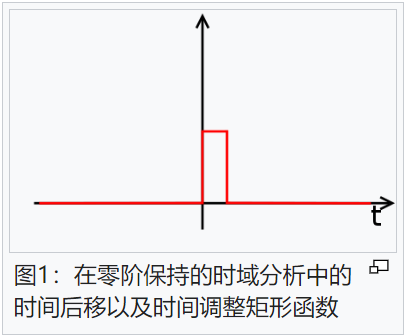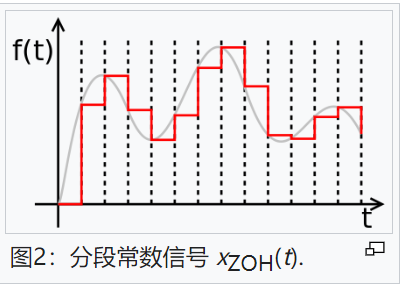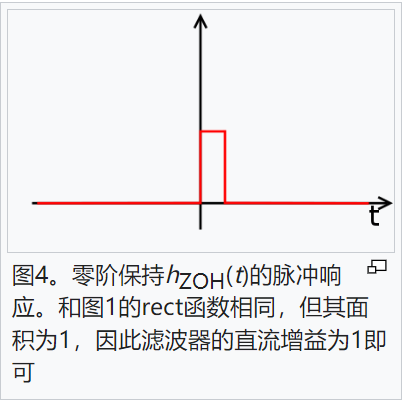400-088-6921155-8888-6921#### 产品分类# 零阶保持

零阶保持（zero-order hold）简称ZOH，是传统数位模拟转换器（DAC）上信号重建的数学模型。此作法会在各取样区间之间，让信号维持之前的值，以此方式将离散信号转换为连续信号，在电子通讯上有许多的应用。

## 时域模型

.零阶保持可以从取样数列x[n]重建为以下的连续时间信号，假设每一个取样的时间间隔都是T：

{\displaystyle x_{\mathrm{ZOH}}(t)\=\sum _{n=-\infty}^{\infty}x[n]\cdot\mathrm{rect}\left({\frac{t-T/2-nT}{T}}\right)\,}{\displaystyle x_{\mathrm{ZOH}}(t)\=\sum _{n=-\infty}^{\infty}x[n]\cdot\mathrm{rect}\left({\frac{t-T/2-nT}{T}}\right)\,}

其中{\displaystyle\mathrm{rect}(),}{\displaystyle\mathrm{rect}(),}为矩形函数。

函数{\displaystyle\mathrm{rect}\left({\frac{t-T/2}{T}}\right)}{\displaystyle\mathrm{rect}\left({\frac{t-T/2}{T}}\right)}如图1所示，而{\displaystyle x_{\mathrm{ZOH}}(t)\,}{\displaystyle x_{\mathrm{ZOH}}(t)\,}是分段常数函数，如图2所示。## 频域模型

上述ZOH输出的方程式也可以表示为冲激响应分段常数函数（rect函数）的线性时不变滤波器之输出，输入则是狄拉克δ函数乘以取样数值所产生的脉冲序列。滤波器可以在频域下进行分析，和其他的信号重建方式进行比较，例如依采样定理建议的惠特克-香农插值公式，或是在二个取样点之间线性内插的一阶保持。

在此作法中，会将狄拉克δ函数的脉冲序列xs(t)经过低通滤波器还原为连续信号x(t)。

虽然实际的数位类比转换器（DAC）不是以此方式进行，不过其其特性可以建模为将假想脉冲序列xs(t)用LTI滤波后所得的特性，而此滤波器的特性是每一个输入脉冲都可以产生持续到下一个取样点的常数步阶输出。

一开始先从取样讯号，配合delta函数建立连续讯号：

{\displaystyle{\begin{aligned}x_{s}(t)&=\sum _{n=-\infty}^{\infty}x[n]\cdot\delta\left({\frac{t-nT}{T}}\right)\\&{}=T\sum _{n=-\infty}^{\infty}x[n]\cdot\delta(t-nT).\end{aligned}}}{\displaystyle{\begin{aligned}x_{s}(t)&=\sum _{n=-\infty}^{\infty}x[n]\cdot\delta\left({\frac{t-nT}{T}}\right)\\&{}=T\sum _{n=-\infty}^{\infty}x[n]\cdot\delta(t-nT).\end{aligned}}}

其中T的比例是因为将delta函数配合时间调整比例而产生的，其意思是使xs(t)的平均值等于在取样的数值，因此低通滤波器的直流增益设定为1即可。有些文献使用这种比例调整方式，不过许多文献不考虑delta函数的系数'T，因此低通滤波器会有一个直流增益T，也就会随取样时间而变化。零阶保持是假想的滤波器或线性系统，可以将调变后的迪拉克脉冲xs(t)转换为片段连续的讯号（如图2）。

{\displaystyle x_{\mathrm{ZOH}}(t)\,=\sum _{n=-\infty}^{\infty}x[n]\cdot\mathrm{rect}\left({\frac{t-nT}{T}}-{\frac{1}{2}}\right)\}{\displaystyle x_{\mathrm{ZOH}}(t)\,=\sum _{n=-\infty}^{\infty}x[n]\cdot\mathrm{rect}\left({\frac{t-nT}{T}}-{\frac{1}{2}}\right)\}

其等效的冲激响应（如图4）为：

{\displaystyle h_{\mathrm{ZOH}}(t)\,={\frac{1}{T}}\mathrm{rect}\left({\frac{t}{T}}-{\frac{1}{2}}\right)={\begin{cases}{\frac{1}{T}}&{\mbox{if}}0\leq t&lt;T\\0&{\mbox{otherwise}}\end{cases}}\}{\displaystyle h_{\mathrm{ZOH}}(t)\,={\frac{1}{T}}\mathrm{rect}\left({\frac{t}{T}}-{\frac{1}{2}}\right)={\begin{cases}{\frac{1}{T}}&{\mbox{if}}0\leq t&lt;T\\0&{\mbox{otherwise}}\end{cases}}\}

其等效频率响应为冲激响应的傅里叶变换。

{\displaystyle H_{\mathrm{ZOH}}(f)\,={\mathcal{F}}\{h_{\mathrm{ZOH}}(t)\}\,={\frac{1-e^{-i2\pi fT}}{i2\pi fT}}=e^{-i\pi fT}\mathrm{sinc}(fT)\}{\displaystyle H_{\mathrm{ZOH}}(f)\,={\mathcal{F}}\{h_{\mathrm{ZOH}}(t)\}\,={\frac{1-e^{-i2\pi fT}}{i2\pi fT}}=e^{-i\pi fT}\mathrm{sinc}(fT)\}

其中{\displaystyle\mathrm{sinc}(x)\}{\displaystyle\mathrm{sinc}(x)\}是正规化的Sinc函数{\displaystyle{\frac{\sin(\pi x)}{\pi x}}}{\displaystyle{\frac{\sin(\pi x)}{\pi x}}}，常用在数位信号处理中。

ZOH的传递函数拉普拉斯变换可以用将s替代为i 2πf而得

{\displaystyle H_{\mathrm{ZOH}}(s)\,={\mathcal{L}}\{h_{\mathrm{ZOH}}(t)\}\,={\frac{1-e^{-sT}}{sT}}\}{\displaystyle H_{\mathrm{ZOH}}(s)\,={\mathcal{L}}\{h_{\mathrm{ZOH}}(t)\}\,={\frac{1-e^{-sT}}{sT}}\}

实际的数位类比转换器（DAC）不会输出狄拉克δ函数的序列xs(t)（因此，若是理想的低通滤波，会在取样前得到独特的带宽受限制的讯号），会输出方波的序列xZOH(t)（阶跃函数），因此ZOH在DAC的频率响应中会有一个本质造成的影响，在频率较高时，会有轻微的信号衰减（在奈奎斯特频率处降低3.9224 dB，对应sinc(1/2)=2/π）。此衰减是因为传统DAC的“保持”特性，不是因为在传统类比数位转换器前面的取样保持电路的影响。

### 相关新闻

##### 联系方式

15588886921

400热线

400-0886921

2466458158@qq.com

##### 二维码